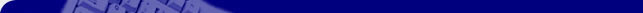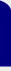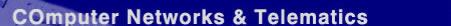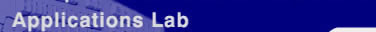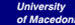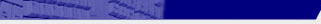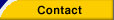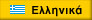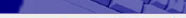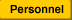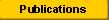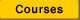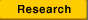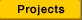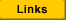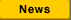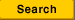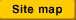A unified game-theoretic methodology for the joint load sharing, routing and congestion control problem Anastasios A. EconomidesPh.D. Thesis, University of Southern California, 1990 This was the first research to formulate, model and solve routing (load balancing, load sharing) problems in computer and communication networks both as Nash games (IEEE ITS 1990,and IEEE Infocom 1991) and as Stackelberg games (Allerton 1990) Abstract (Summary) In this dissertation, we introduce a unified game-theoretic methodology for the multi-objective joint load sharing, routing and congestion control problem in distributed systems. We further propose stochastic learning automata algorithms for decentralized asynchronous dynamic computation of the solution. First, we define and model the problem on the path flow space using queueing and state space models. Then we develop three methodologies for both the quasi-static and the dynamic causes of the problem: (i) Team optimization methodology, when the classes of jobs cooperate for the socially optimum, (ii) Nash game methodology, when the classes of jobs compete among themselves and each class tries to operate optimally for its own jobs, and (iii) Stackelberg game methodology, when some classes of jobs have more power than others, for example priority classes. For each methodology, we formulate the problem as a Nonlinear Programming, an Optimal Control, a Dynamic Programming, a Nonlinear Complementarity and a Variational Inequality problem. We state conditions for existence/uniqueness of the solution, and derive the optimality conditions for the quasi-static problem using the Karush-Kuhn-Tucker theorem, and for the dynamic problem using Pontryagin's maximum principle. We apply the proposed methodologies to Datagram, Virtual Circuit and Integrated Services Networks and develop several new queueing models and performance measures, for each network type. We explicitly solve several examples and evaluate the system performance via simulation. Finally, we propose decentralized asynchronous dynamic load sharing, routing and congestion control using Stochastic Learning Automata. We introduce new classes of Stochastic Learning Automata algorithms and use them as decision makers in the distributed system. We explicitly illustrate their operation with an example for dynamic virtual circuit routing and demonstrate via simulation improved system performance. Download the Ph.D. Thesis: Download one large PDF document (approx. 80MB) Download Volume I (approx. 36MB) and Volume II (approx. 40MB) separatelyÁń÷Ţ óĺëßäáň(c) 2001 created by Magnet Internet Services